• 什么是JMH？
• 创建 JMH 基准
• 运行基准测试
• 使用 IDE 插件
• JMH 基准参数
• JMH 使用示例

# Technical requirements

• 装有 Microsoft Windows、Apple macOS 或 Linux 操作系统的计算机
• Java SE 版本 17 或更高版本
• 您喜欢的 IDE 或代码编辑器

# What is JMH?

``class SomeClass {``
``    public int someMethod(int m, int s) {``
``        int res = 0;``
``        for(int i = 0; i < m; i++){``
``            int n = i * i;``
``            if (n != 0 && n % s == 0) {``
``                res =+ n;``
``            }``
``        }``
``        return res;``
``    }``
``}``

``public void testCode() {``
``   StopWatch stopWatch = new StopWatch();``
``   stopWatch.start();``
``   int xN = 100_000;``
``   int m = 1000;``
``   for(int x = 0; i < xN; x++) {``
``        int res = 0;``
``        for(int i = 0; i < m; i++){``
``            int n = i * i;``
``            if (n != 0 && n % 250_000 == 0) {``
``                res += n;``
``            }``
``        }``
``    }``
``    System.out.println("Average time = " + ``
``           (stopWatch.getTime() / xN /m) + "ms");``
``}``

``public int testCode() {``
``   StopWatch stopWatch = new StopWatch();``
``   stopWatch.start();``
``   int xN = 100_000;``
``   int m = 1000;``
``   int res = 0;``
``   for(int x = 0; i < xN; x++) {``
``        for(int i = 0; i < m; i++){``
``            int n = i * i;``
``            if (n != 0 && n % 250_000 == 0) {``
``                res += n;``
``            }``
``        }``
``   }``
``   System.out.println("Average time = " + ``
``          (stopWatch.getTime() / xN / m) + "ms");``
``   return res;``
``}``

``public void testCode() {``
``   StopWatch stopWatch = new StopWatch();``
``   stopWatch.start();``
``   int xN = 100_000;``
``   int m = 1000;``
``   SomeClass someClass = new SomeClass();``
``   for(int x = 0; i < xN; x++) {``
``        someClass.someMethod(m, 250_000);``
``    }``
``    System.out.println("Average time = " + ``
``          (stopWatch.getTime() / xN / m) + "ms");``
``}``

# Creating a JMH benchmark

``<dependency>``
``    <groupId>org.openjdk.jmh</groupId>``
``    <artifactId>jmh-core</artifactId>``
``    <version>1.21</version>``
``</dependency>``
``<dependency>``
``    <groupId>org.openjdk.jmh</groupId>``
``    <artifactId>jmh-generator-annprocess</artifactId>``
``    <version>1.21</version>``
``</dependency>``

``public class BenchmarkDemo {``
``    public static void main(String... args) throws Exception{``
``        org.openjdk.jmh.Main.main(args);``
``    }``
``    @Benchmark``
``    public void testTheMethod() {``
``        int res = 0;``
``        for(int i = 0; i < 1000; i++){``
``            int n = i * i;``
``            if (n != 0 && n % 250_000 == 0) {``
``                res += n;``
``            }``
``        }``
``    }``
``}``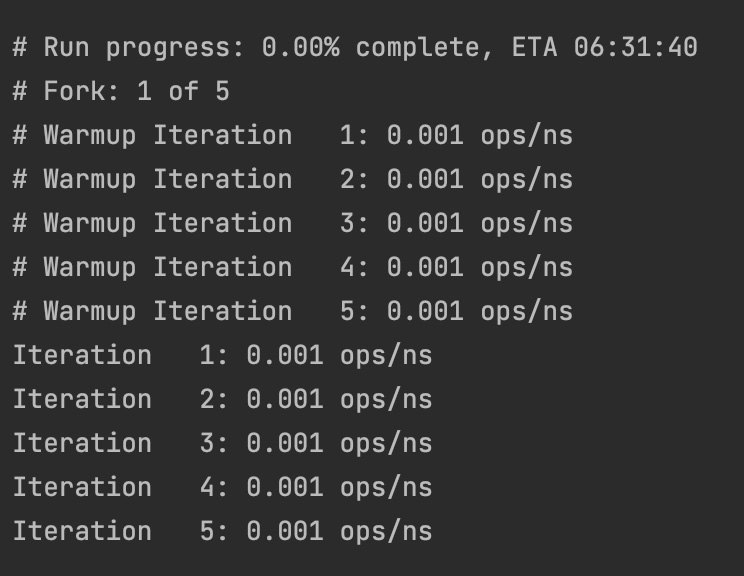# Running the benchmark

## Using an IDE plugin

1. 要开始安装插件，请同时按下 命令 键和 逗号 (,) 单击顶部水平菜单中的扳手符号（带有悬停文本 首选项）：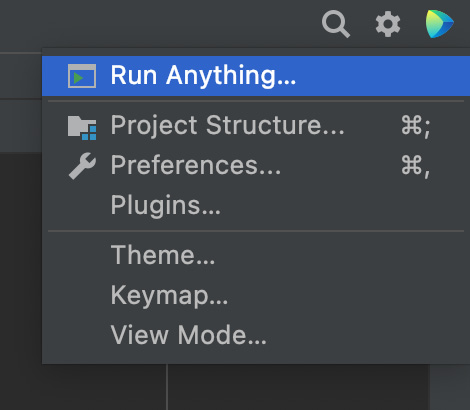1. 它将在左窗格中打开一个带有以下菜单的窗口：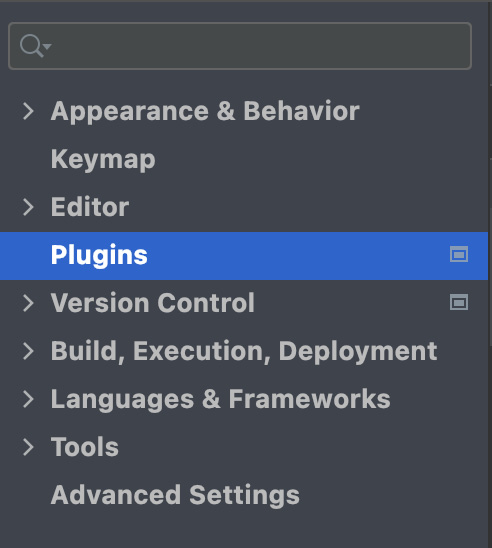1. 选择 Plugins，如前面截图所示， 观察  ;具有以下顶部水平菜单的窗口：1. 选择 Marketplace，输入 `JMH` 在 Marketplace中的搜索插件 strong> 输入字段，然后按 Enter。如果您有互联网连接，它将显示一个 JMH 插件 符号，类似于以下屏幕截图中显示的符号：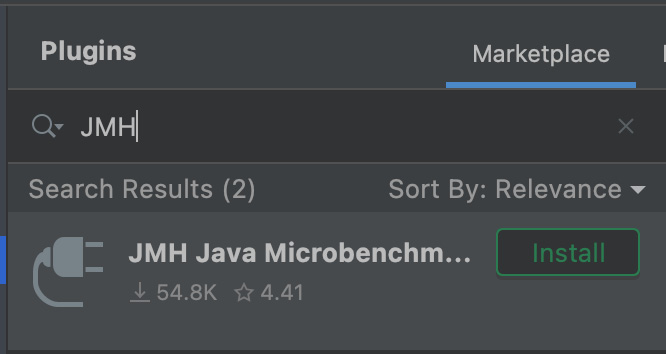1. 点击 安装 按钮 和然后，变成 重启IDE ， 再次点击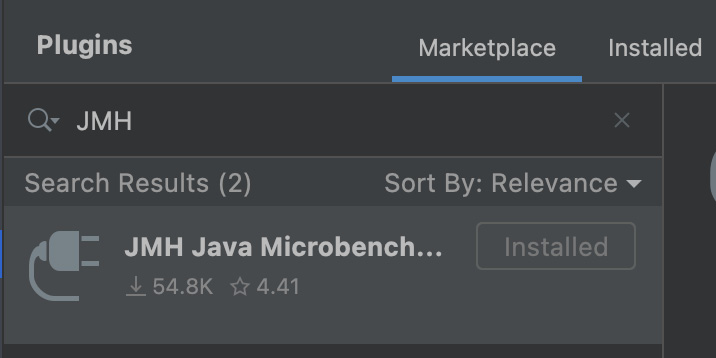1. IDE 重启后，插件就可以使用了。现在，您不仅可以运行 `main()` 方法 还可以选择 如果你有几个 方法要执行的基准方法 `@Benchmark` 注解。为此，请从 运行 下拉菜单中选择 运行...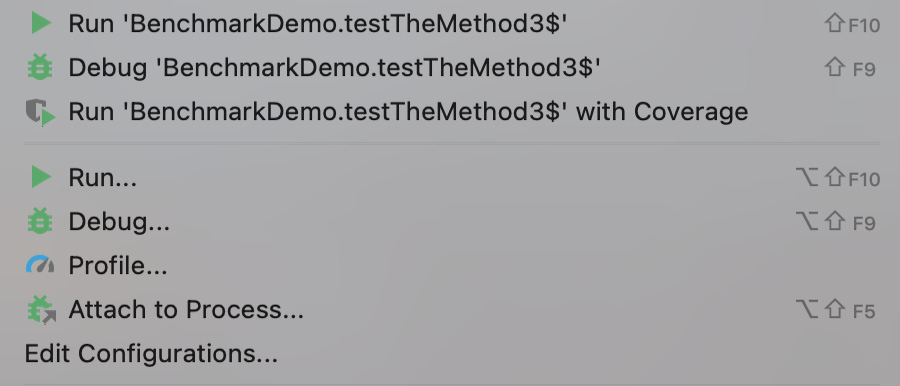1. 它将带来 一个窗口，其中包含您可以运行的一系列方法：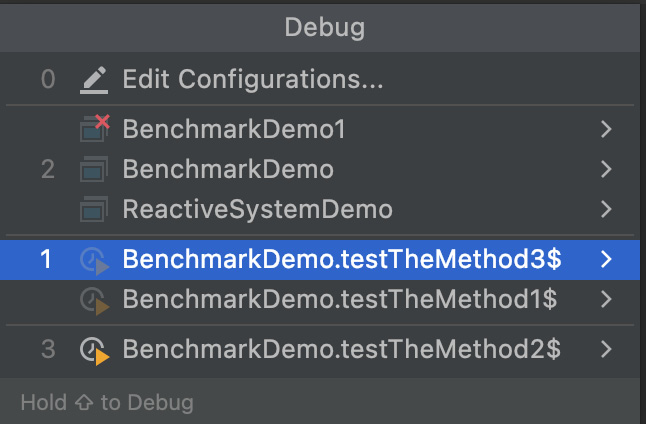1. 选择您要运行的那个，它将被执行。至少运行一次方法后，只需右键单击它并从弹出菜单中执行它：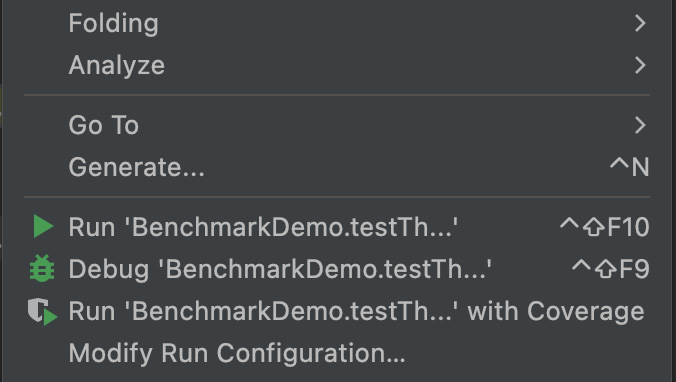1. 您还可以使用每个菜单项右侧显示的快捷方式。

# JMH benchmark parameters

## Mode

• `Mode.AverageTime`：测量平均执行时间
• `Mode.Throughput`：通过在迭代中调用基准方法来测量吞吐量
• `Mode.SampleTime`：对执行时间进行采样，而不是对其进行平均； 允许我们推断分布、百分位数等
• `Mode.SingleShotTime`：测量单个方法 调用 时间; 允许在不连续调用基准方法的情况下进行冷启动测试

``@BenchmarkMode(Mode.AverageTime)``

``@BenchmarkMode({Mode.Throughput, Mode.AverageTime, Mode.SampleTime, Mode.SingleShotTime}``

``@BenchmarkMode(Mode.All)``

## Output time unit

``@OutputTimeUnit(TimeUnit.NANOSECONDS)``

## Iterations

``@Warmup(iterations = 5, time = 100, ``
``                          timeUnit =  TimeUnit.MILLISECONDS)``
``@Measurement(iterations = 5, time = 100, ``
``                           timeUnit = TimeUnit.MILLISECONDS)``

## Forking

``@Fork(10)``

`warmups` 参数是 另一个可以设置 指示基准必须执行多少次而不收集测量值的参数：

``@Fork(value = 10, warmups = 5)``

``@Fork(value = 10, jvmArgs = {"-Xms2G", "-Xmx2G"})``

JMH 参数的完整列表以及如何使用它们的示例可以在 `openjdk` project (http://hg.openjdk.java.net/code -tools/jmh/file/tip/jmh-samples/src/main/java/org/openjdk/jmh/samples）。比如我们没有提到 `@Group``@GroupThreads`` @Measurement``@Setup``@Threads``@Timeout`、 `@TearDown` 或 `@Warmup`

# JMH usage examples

``@Benchmark``
``@BenchmarkMode(Mode.All)``
``@OutputTimeUnit(TimeUnit.NANOSECONDS)``
``public void testTheMethod0() {``
``    int res = 0;``
``    for(int i = 0; i < 1000; i++){``
``        int n = i * i;``
``        if (n != 0 && n % 250_000 == 0) {``
``            res += n;``
``        }``
``    }``
``}``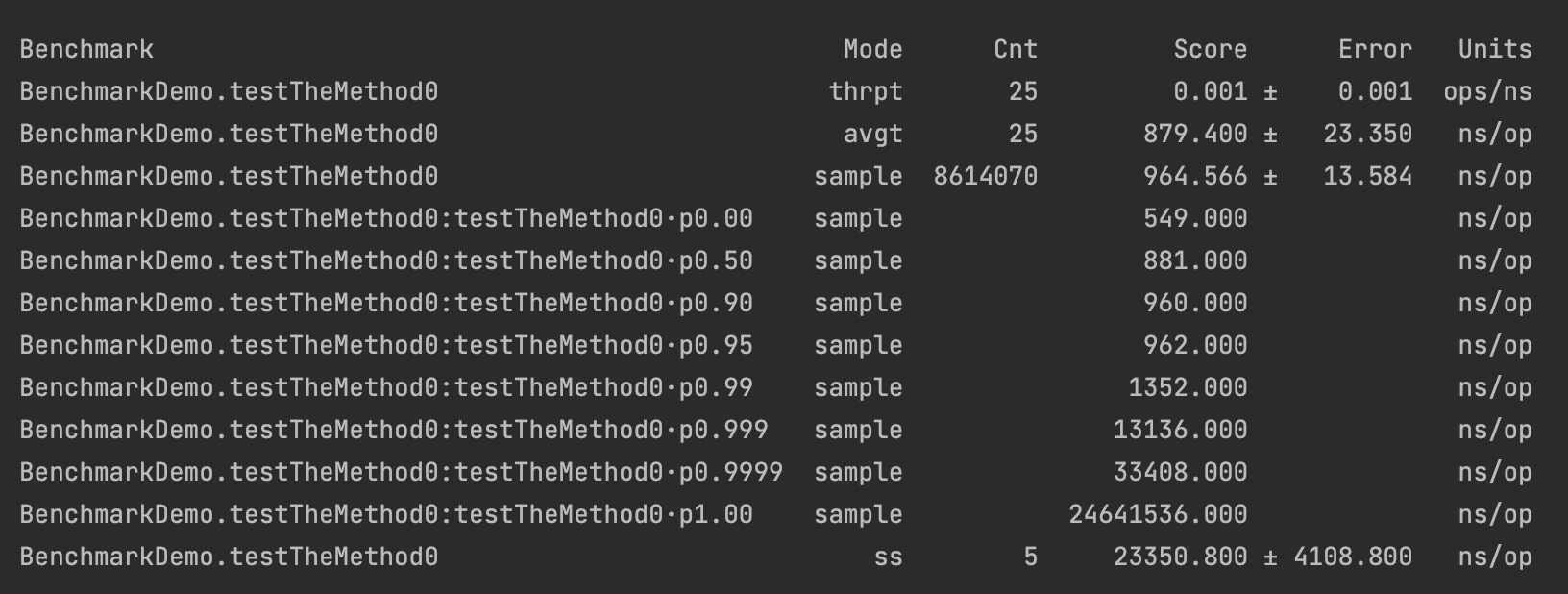``@Benchmark``
``@BenchmarkMode(Mode.All)``
``@OutputTimeUnit(TimeUnit.NANOSECONDS)``
``public void testTheMethod1() {``
``    SomeClass someClass = new SomeClass();``
``    int i = 1000;``
``    int s = 250_000;``
``    someClass.someMethod(i, s);``
``}``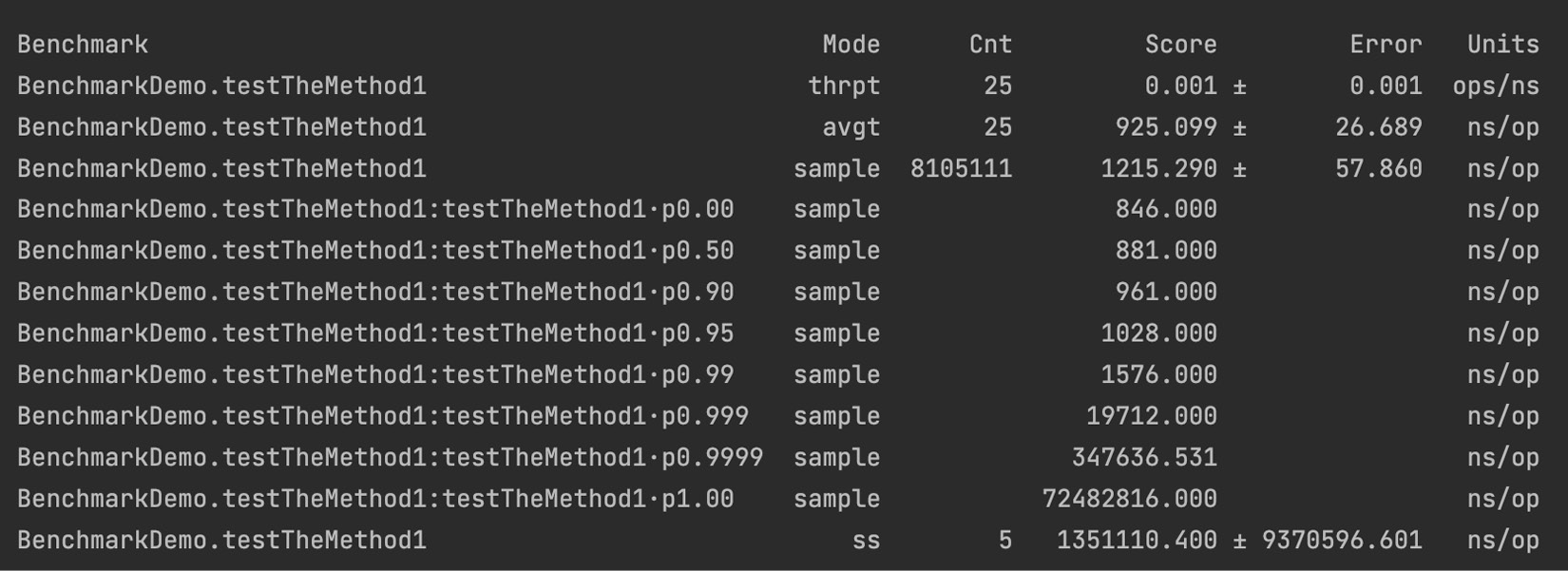## Using the @State annotation

``@State(Scope.Thread)``
``public static class TestState {``
``    public int m = 1000;``
``    public int s = 250_000;``
``}``
``@Benchmark``
``@BenchmarkMode(Mode.All)``
``@OutputTimeUnit(TimeUnit.NANOSECONDS)``
``public int testTheMethod3(TestState state) {``
``    SomeClass someClass = new SomeClass();``
``    return someClass.someMethod(state.m, state.s);``
``}``

`Scope` 值用于在测试之间共享数据。在我们的例子中，只有一个使用 `TestCase` 类对象的测试，我们不需要共享。否则，该值可以设置为 `Scope.Group` 或 `Scope.Benchmark`，这意味着我们可以添加setter到 `TestState` 类并在其他测试中读取/修改它。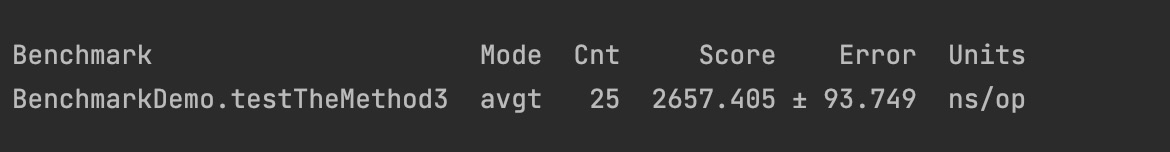## Using the Blackhole object

``@Benchmark``
``@BenchmarkMode(Mode.All)``
``@OutputTimeUnit(TimeUnit.NANOSECONDS)``
``public void testTheMethod4(TestState state, ``
``                                       Blackhole blackhole){``
``  SomeClass someClass = new SomeClass();``
``  blackhole.consume(someClass.someMethod(state.m, state.s));``
``}``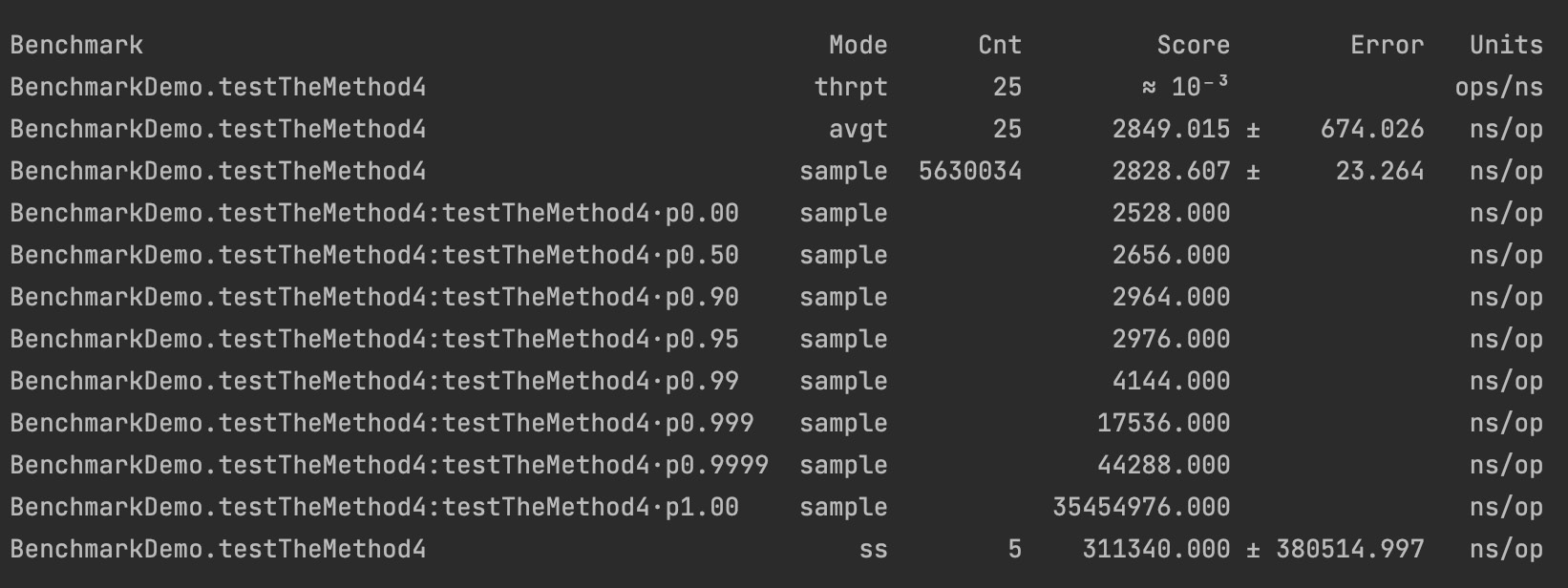## Using the @CompilerControl annotation

``class SomeClass{``
``     public int oneMethod(int m, int s) {``
``        int res = 0;``
``        for(int i = 0; i < m; i++){``
``            int n = i * i;``
``            if (n != 0 && n % s == 0) {``
``                res = anotherMethod(res, n);``
``            }``
``        }``
``        return res;``
``    }``
``    @CompilerControl(CompilerControl.Mode.EXCLUDE)``
``    private int anotherMethod(int res, int n){``
``        return res +=n;``
``    }``
``}``

• `Mode.INLINE`：强制方法内联
• `Mode.DONT_INLINE`: 避免方法内联
• `Mode.EXCLUDE`: To避免方法编译

## Using the @Param annotation

`@Param` 是各种框架使用的标准Java注解，例如JUnit。它标识一组参数值。带有 `@Param` 注解的测试将运行与数组中的值一样多的次数。 每次测试执行都会从数组中获取不同的值。

``@State(Scope.Benchmark)``
``public static class TestState1 {``
``    @Param({"100", "1000", "10000"})``
``    public int m;``
``    public int s = 250_000;``
``}``
``@Benchmark``
``@BenchmarkMode(Mode.All)``
``@OutputTimeUnit(TimeUnit.NANOSECONDS)``
``public void testTheMethod6(TestState1 state, ``
``                                       Blackhole blackhole){``
``  SomeClass someClass = new SomeClass();``
``  blackhole.consume(someClass.someMethod(state.m, state.s));``
``}``

`testTheMethod6()` benchmark 是 going与每个列出的参数值 `m`

# A word of caution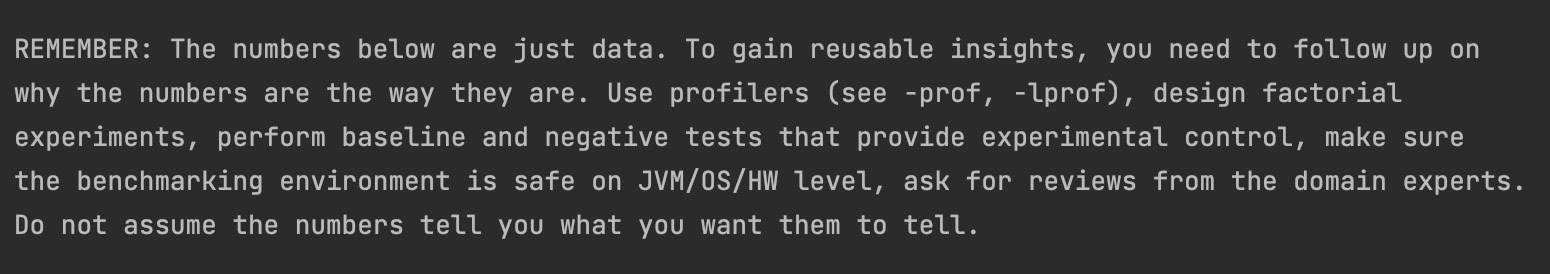# Quiz

1. 选择所有正确的陈述：
1. JMH 毫无用处，因为 它在生产环境之外运行方法。
2. JMH 能够解决一些 JVM 优化问题。
3. JMH 不仅可以测量平均性能时间 还可以测量其他性能特征。
4. JMH 也可用于衡量小型应用程序的性能。
2. 列出开始使用 JMH 所需的两个步骤。
3. 说出 JMH 可以运行的四种方式。
4. 列出可以与 JMH 一起使用（测量）的两种模式（性能特征）。
5. 列出两个可用于呈现 JMH 测试结果的时间单位。
6. 如何在 JMH 基准测试之间共享数据（结果、状态）？
7. 您如何告诉 JMH 使用枚举值列表运行参数的基准测试？
8. 如何强制或关闭方法的编译？
9. JVM的不断折叠优化怎么关闭？
10. 如何以编程方式提供 Java 命令选项以运行特定的基准测试？